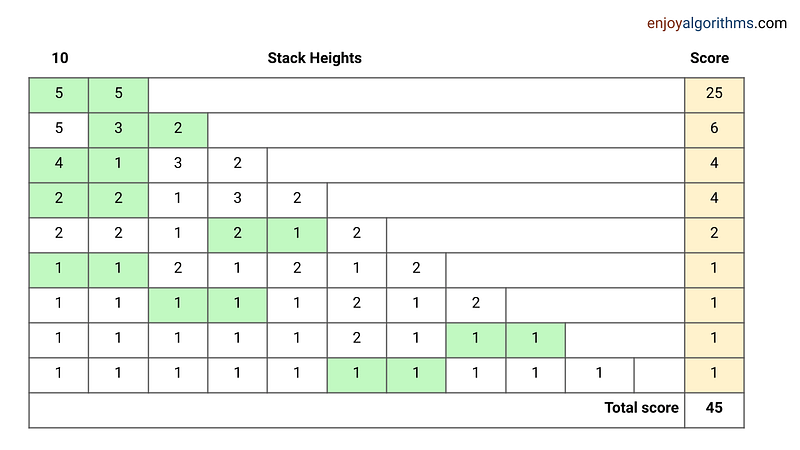# Box Unstacking Problem

Related tags:

algorithmic-puzzles

### Problem Statement

You begin with a stack of n boxes. Then you make a sequence of moves. In each move, you divide one stack of boxes into two non-empty stacks. The game ends when you have n stacks, each containing a single box.

You earn points for each move. For example, if you divide one stack of heights A + B into two stacks of heights A and B, you will score AB points for that move. The overall score is the sum of the points that you earn for each move. (A sample example is given in the image below).

What strategy should you use to maximize your total score? For example, suppose we begin with a stack of n = 10 boxes. Here is one possible way to proceed with the game:Here is the most interesting part! Prove that: Any strategy of unstacking n boxes will give a score of n(n − 1)/2 points. In other words: Prove that the overall score will depend entirely on the number of boxes, irrelevant to what strategy you choose.

### Proof using induction

Suppose P(n) is the total points achieved after unstacking n boxes. Now we need to prove that P(n) = n(n − 1)/2. One idea is to use induction. In this case, we prove that base case P(1) is true and P(1), . . . , P(n − 1) imply P(n) for all n ≥ 2.

#### Base case

If n = 1, then there is only one box and no moves are possible. So total points = 1(1 − 1)/2 = 0 and P(1) is true.

#### Inductive steps

We have a stack of n boxes. In the first move, suppose we split n boxes into two substacks with sizes k and n − k (0< k < n). Now total points for the game is the sum of points for the first move plus points obtained by unstacking the two smaller substacks.

=> Total points for unstacking n boxes = (Points for 1st move) + (Points for unstacking k boxes) + (Points for unstacking n − k boxes)

• We are dividing n boxes into two substacks of size k and n — k. So total points for the 1st move = k(n − k).
• If P(n) is the point for unstacking n boxes, then points for unstacking k boxes = P(k), and Points for unstacking n − k boxes = P(n — k).

We put these values in the above equation:

=> P(n) = k(n − k) + P(k) + P(n — k)

Now for the inductive step, suppose P(1), P(2)… P(n — 2), P(n — 1) are true i.e. P(k) = k(k − 1)/2, where 0 < k < n. Now we need to prove that P(n) will be also true.

So based on the above assumption:

• P(k) = k(k − 1)/2
• P(n — k) = (n − k)(n − k − 1)/2

=> P(n) = k(n − k) + k(k − 1)/2 + (n − k)(n − k − 1)/2

= (2nk − 2k² + k² − k + n² − nk − n − nk + k² + k)/2

= n(n − 1)/2 (Several terms cancel out each other.)

So if P(1), P(2)… P(n — 2), and P(n — 1) are true, then P(n) is also true. Similarly, we can prove that P(n + 1) will be also true, if P(1), P(2), . . . P(n) are true. And so on!

A critical question to think: Instead of using induction, can we think of solving this recurrence [P(n) = k(n — k) + P(k) + P(n — k)] directly to obtain the value n(n — 1)/2? What challenges are associated with solving this recurrence?

Enjoy learning, enjoy mathematics!

Share on social media:

© 2020 Code Algorithms Pvt. Ltd.